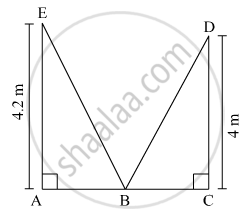Advertisement Remove all ads

# Walls of Two Buildings on Either Side of a Street Are Parellel to Each Other. a Ladder 5.8 M Long is Placed on the Street Such that Its Top Just Reaches the Window of a Building at the Height of 4 M. - Geometry

Walls of two buildings on either side of a street are parellel to each other. A ladder 5.8 m long is placed on the street such that its top just reaches the window of a building at the height of 4 m. On turning the ladder over to the other side of the street , its top touches the window of the other building at a height 4.2 m. Find the width of the street.

Advertisement Remove all ads

#### SolutionLet the length of the ladder be 5.8 m.
According to Pythagoras theorem, in ∆EAB

${EA}^2 + {AB}^2 = {EB}^2$
$\Rightarrow \left( 4 . 2 \right)^2 + {AB}^2 = \left( 5 . 8 \right)^2$
$\Rightarrow 17 . 64 + {AB}^2 = 33 . 64$
$\Rightarrow {AB}^2 = 33 . 64 - 17 . 64$
$\Rightarrow {AB}^2 = 16$
$\Rightarrow AB = 4 m . . . \left( 1 \right)$

In ∆DCB

${DC}^2 + {CB}^2 = {DB}^2$
$\Rightarrow \left( 4 \right)^2 + {CB}^2 = \left( 5 . 8 \right)^2$
$\Rightarrow 16 + {CB}^2 = 33 . 64$
$\Rightarrow {CB}^2 = 33 . 64 - 16$
$\Rightarrow {CB}^2 = 17 . 64$
$\Rightarrow CB = 4 . 2 m . . . \left( 2 \right)$

From (1) and (2), we get

$AB + BC = \left( 4 + 4 . 2 \right) m$
$= 8 . 2 m$

Hence, the width of the street is 8.2 m.

Is there an error in this question or solution?
Advertisement Remove all ads

#### APPEARS IN

Balbharati Mathematics 2 Geometry 10th Standard SSC Maharashtra State Board
Chapter 2 Pythagoras Theorem
Practice Set 2.1 | Q 10 | Page 39
Advertisement Remove all ads
Advertisement Remove all ads
Share
Notifications

View all notifications

Forgot password?